# 单目视觉定位测距的两种方式

单目定位和双目定位的选择，我觉得主要还是成本和时间的考虑。之前也尝试过双目定位，感觉要更精准些，但双目测距需要对两幅图像进行图像变换和极线匹配，稍微耗时了一些。这几天尝试了一下单摄像头进行测距定位，主要有两个思路：
1.定位测量插值得到每个像素的实际坐标
该方法总觉得有很大的问题：一个是摄像头安装后就必须固定不动，稍微的旋转都会导致之间测量的像素点对应的坐标偏移。另一个是人工测量的工程量之大，对于1024*1280像素的摄像头，准确的测量就应该是130万个点，而就算我们按米来分割地面，10*20m2的地面也要测量200个点，就算可以通过算法自动识别，做200个标志就算测量画线也是令人头疼的。考虑到针孔成像模型的等比例放大，我们通过直接打印布满等距阵列圆点的纸来进行测量。
其原理如下：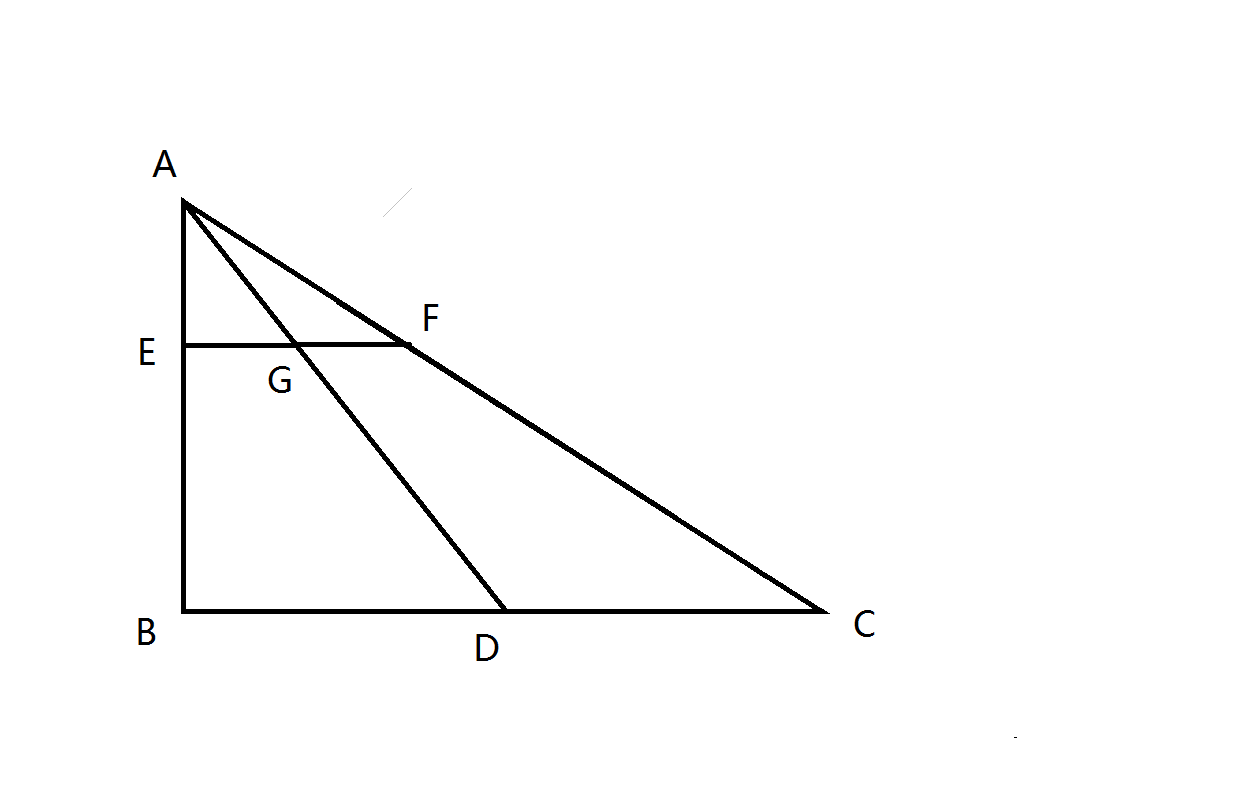根据相似三角形的等比例关系，有:

EGBD=AGAD$\dfrac{EG}{BD}=\dfrac{AG}{AD}$

GFDC=AGAD$\dfrac{GF}{DC}=\dfrac{AG}{AD}$

同时又有：
EGEB=AEAB$\dfrac{EG}{EB}=\dfrac{AE}{AB}$

通过等式传递有：
EGBD=GFDC=AEAB$\dfrac{EG}{BD}=\dfrac{GF}{DC}=\dfrac{AE}{AB}$

如此一来，可以在较高距离测量等距阵列点（如标定板），经过插值，再进行等比例放大即可得到每个像素点对应的实际地面的坐标。

处理的示意图如下：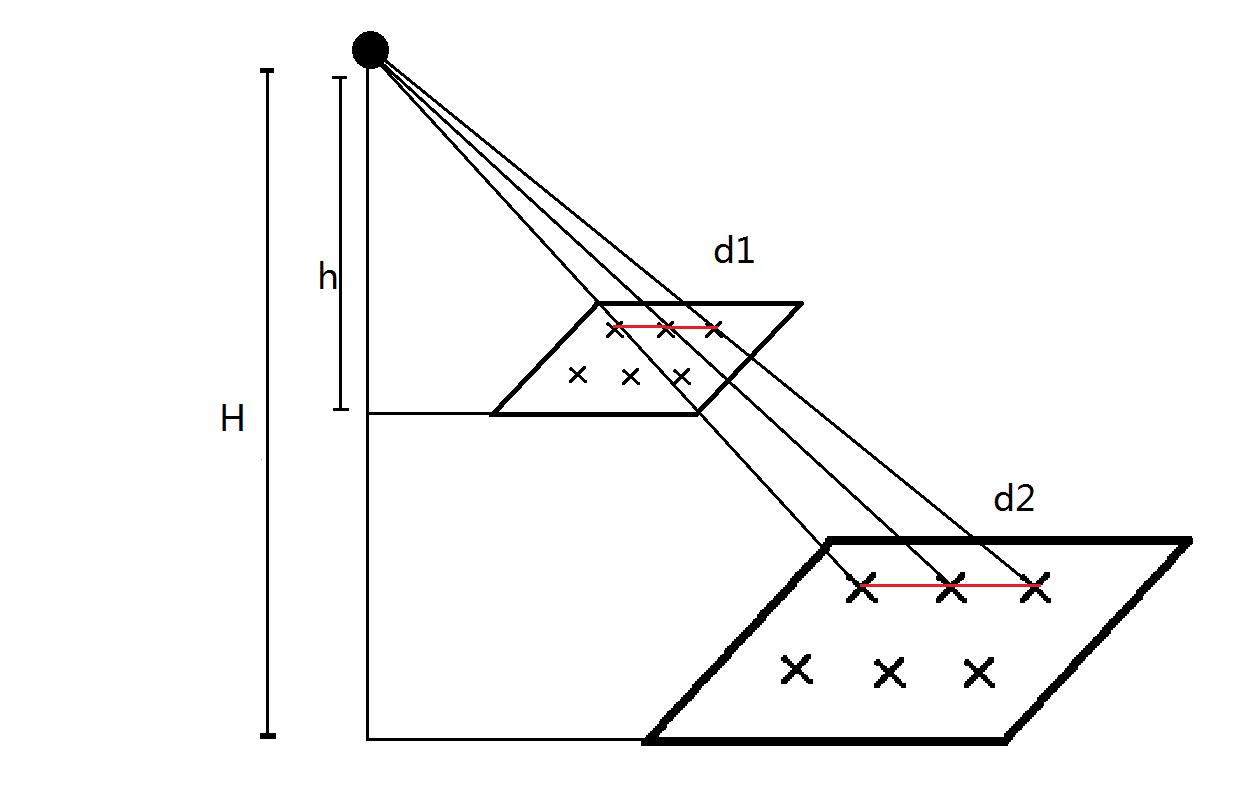这样操作可以省去人工在地面测量绘画标志。测量好纸上的点距后再进行H/h的放大就可以得到像素对应实际地面的坐标。但实际操作过程中遇到的问题是图像上边缘的梯形失真过于严重，导致打印纸上的标志点不容易识别，因此还需要准备不同距离的等距阵列圆点图。

2.根据相似三角比例计算出对应像素点的实际坐标
这个方法对摄像机标定的要求比较高，同时要求镜头本身造成的畸变就比较小，但总体来说这种方法的可移植性和实用性都较强。其主要的思路还是小孔成像的模型。
模型一：假设测量的点都在Y轴上，此时无X轴分量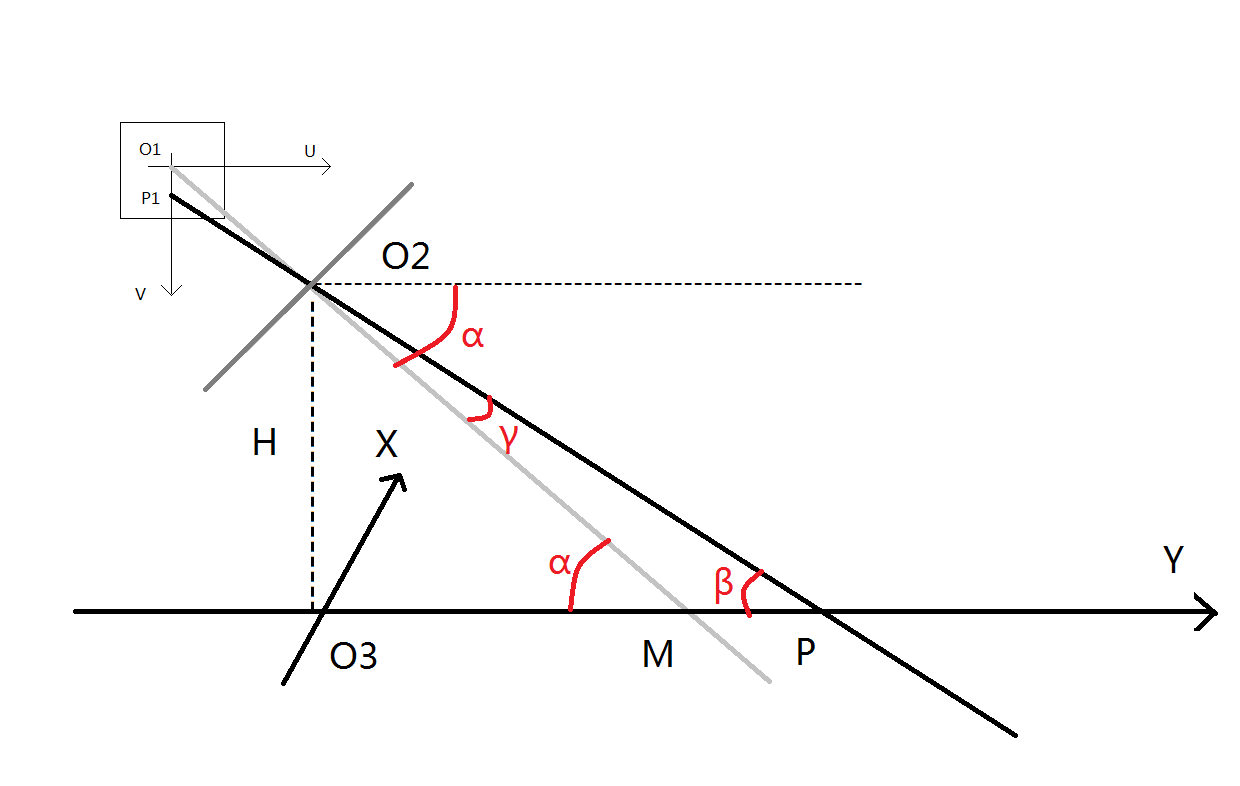该图主要有三个坐标系，分别是图像坐标系UO1V$\small{UO_1V}$，以O2$\small{O_2}$为原点的摄像机坐标系，世界坐标系XO3Y$\small{XO_3Y}$。（相关的内容我就不复述了，不懂的百度和知乎上都有，搜搜相机标定的知识）
我们可以看到，世界坐标中的点通过光轴成像在图像坐标的点是成比例的，其比例媒介就是相机镜头中心在图像上的像素点O1与其在世界坐标中的实际点M，通过推导可以求解O3P$\small{O_3P}$的长度。（注意，由于相机安装一般都有误差，所以镜头中心点不一定是图像的中点，所以ucenter，vcenter不一定为0）

一步步推导如下：
已知量：摄像机高度H
图像坐标中心对应的世界坐标点与摄像头在y轴上的距离O3M$O_{3}M$
镜头中心点的图像坐标(ucentervcenter)$(ucenter，vcenter)$
测量像素点的图像坐标P1(u0$P_1(u，0）$
实际像素的长度xpix
实际像素的宽度ypix。
摄像头焦距f
（镜头中心点图像坐标，焦距，像素长宽都可以由标定直接求解出来，通过halcon的标定助手可以很容易的得到，openCV也有相应的程序）
α=arctan(HO3M)$α=arctan(\frac{H}{\small{O_3M}})$

γ=arctan(O1P1×ypixf)=(vvcenter)ypixf$γ=arctan(\frac{\small{O_1P_1} \times\large{ ypix}}{f})=\frac{\large{(v-vcenter)}*\large{ypix}}{f}$

β=αγ$β=α-γ$

O3P=Htan(β)$\small{O_3P}=\dfrac{H}{tan(β)}$

这样就可以得到垂直方向的坐标　Y=O3P$\small{Y=O_3P}$

模型二：假设测量的点有X轴、Y轴分量

针对以下模型图我们将进行说明：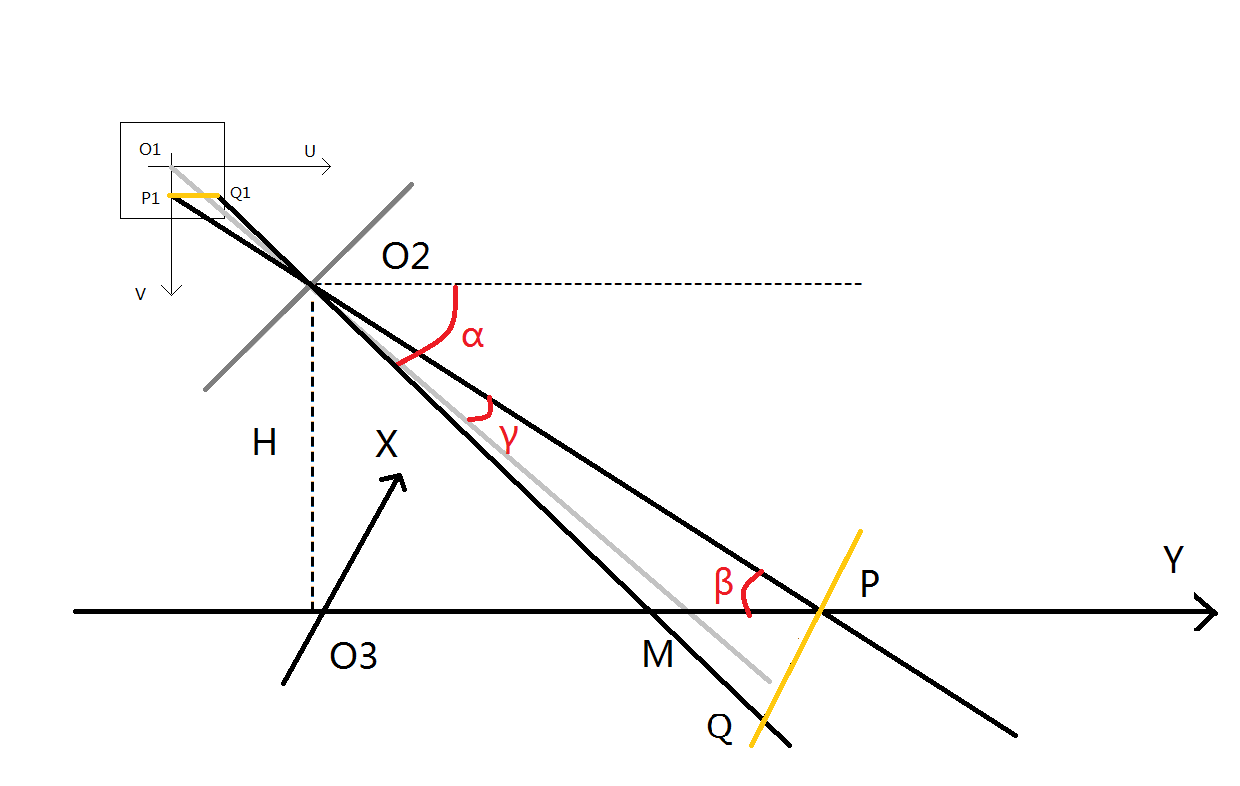一步步推导如下：
已知量：摄像机高度H
图像坐标中心对应的世界坐标点与摄像头在y轴上的距离O3M$O_{3}M$
镜头中心点的图像坐标O1(ucentervcenter)$O_1(ucenter，vcenter)$
测量像素点的图像坐标P1(u0Q1uv$P_1(u，0）、Q_1（u，v）$
实际像素的长度xpix
实际像素的宽度ypix
摄像头焦距f
（y轴方向计算和上一个模型相同，x轴计算是y轴坐标通过比例计算得到）
α=arctan(HO3M)$α=arctan(\frac{H}{\small{O_3M}})$

γ=arctan(O1P1×ypixf)=(vvcenter)ypixf$γ=arctan(\frac{\small{O_1P_1} \times\large{ ypix}}{f})=\frac{\large{(v-vcenter)}*\large{ypix}}{f}$

β=αγ$β=α-γ$

O3P=Htan(β)$\small{O_3P}=\dfrac{H}{tan(β)}$

这样就可以得到垂直方向的坐标　Y=O3P$\small{Y=O_3P}$

O2P1=(vvcenter)xpix)2+f2$\small{O_2P_1}=\sqrt{ (v-vcenter)*xpix)^2+f^{2}}$

O2P=Hsin(β)$\small{O_2P}=\dfrac{H}{sin(β)}$

由PQP1Q1=O2PO2P1$\small{\dfrac{PQ}{P_1Q_1}=\dfrac{O_2P}{O_2P_1}}$　得到　PQ=O2P×P1Q1O2P1$\small{PQ=\dfrac{O_2P\times P_1Q_1}{O2P1}}$

这样就可以得到垂直方向的坐标　X=PQ$\small{X=PQ}$
模型三：假设测量的点有X轴、Y轴分量，且物体有高度h

针对以下模型图我们将进行说明：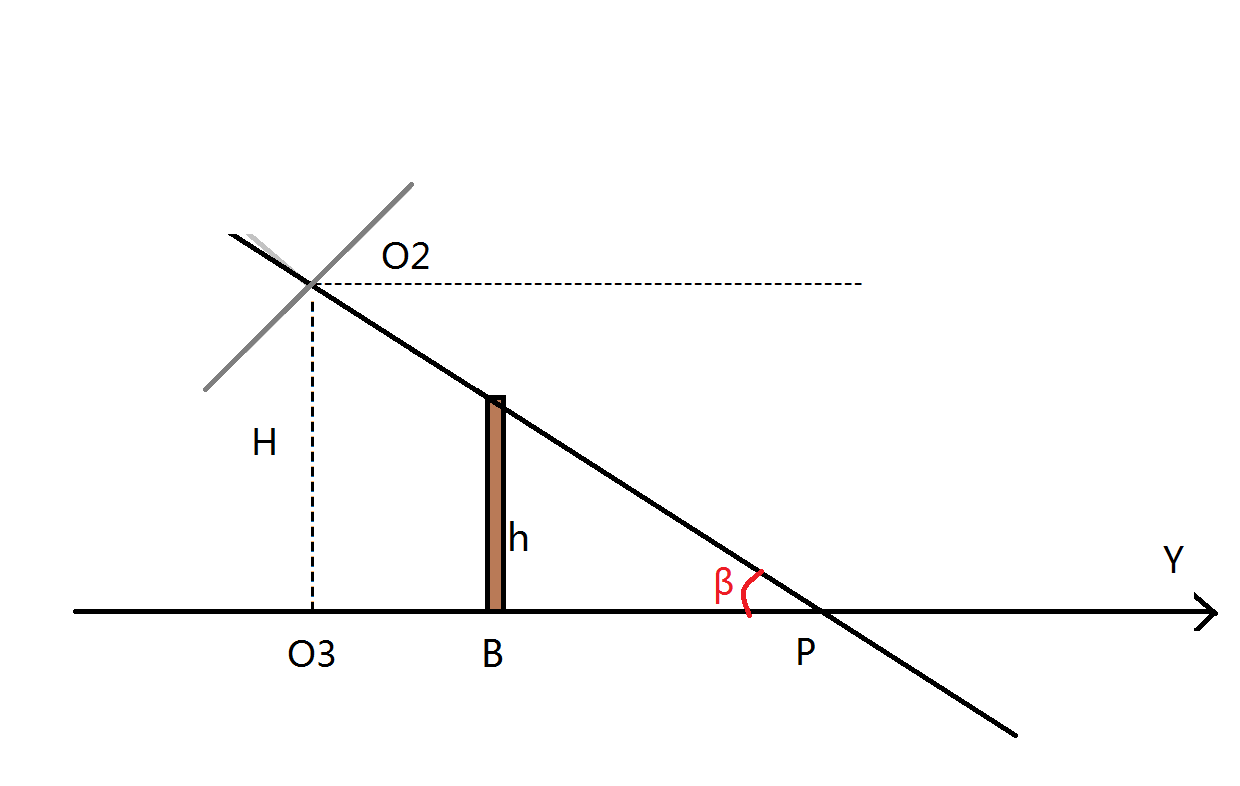由于物体有高度，因此还需要进行一个投影变换，其实也还是相似变换

设真实的坐标(X,Y)$\small{(X',Y')}$，模型二求出的坐标(X,Y)$\small{(X,Y)}$

则(X,Y)=(X,Y)×(1hH)$\small{(X',Y')}=\small{(X,Y)}\times (1-\dfrac{h}{H})$
模型评价

实际操作过程中，发现该模型在畸变较小的图像中误差较小，且在矫正畸变后的图像中测量的图像坐标可以得到更准确的值。另一方面，在实际操作过程中，发现由于镜头有稍微歪曲或者内置感光区域安装歪了，可能导致我们找不到真正的X,Y轴，所以在测量验证的时候会有稍许误差，但即便如此，在10米的定位下，误差也不到5%，效果还是可以接受的。如果有人知道如何找到真正的X,Y轴，请博客留言告知一下，先谢谢了。